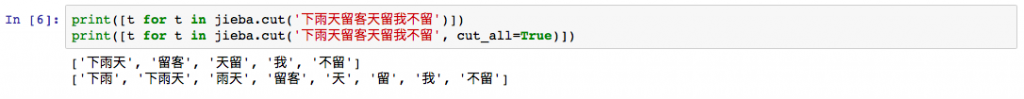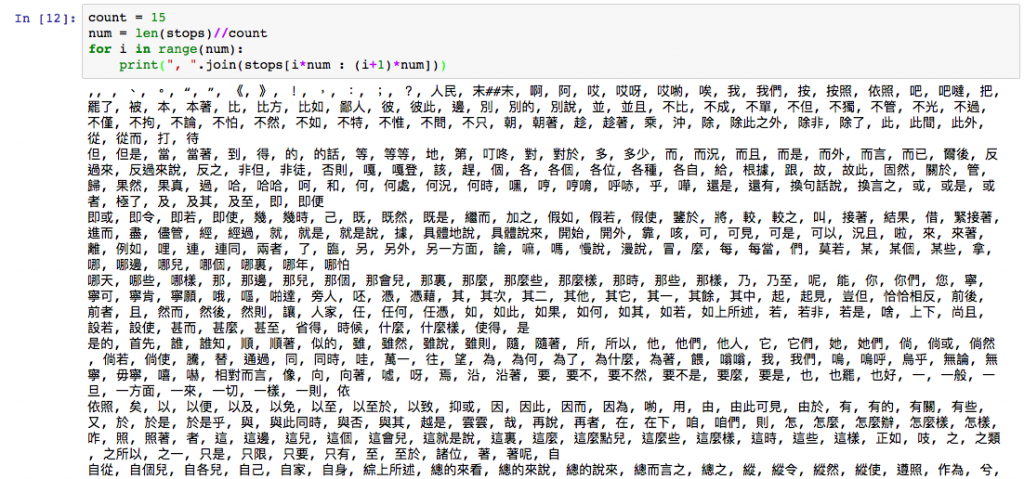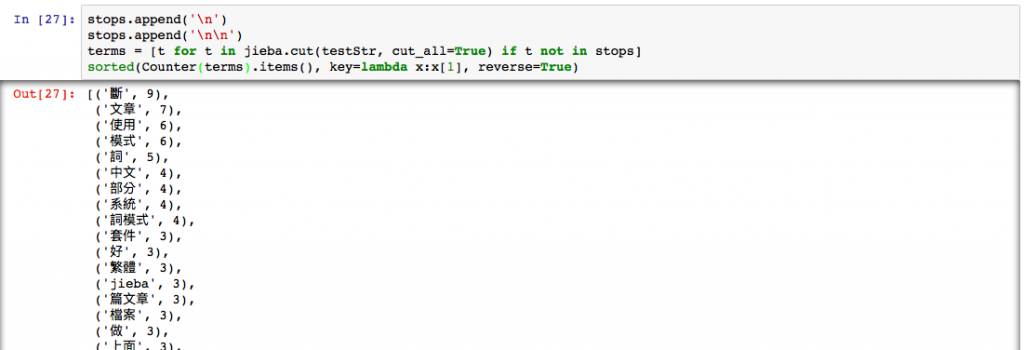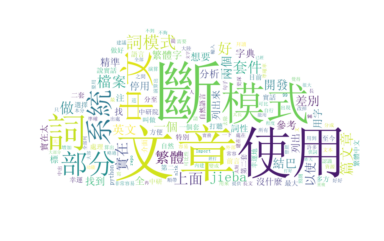#2018 iT 邦幫忙鐵人賽DAY 16
0
AI & Machine Learning

# Import

``````import jieba
jieba.set_dictionary('dict.txt.big')  # 如果是使用繁體文字，請記得去下載繁體字典來使用
with open('stops.txt', 'r', encoding='utf8') as f:  # 中文的停用字，我也忘記從哪裡拿到的，效果還可以，繁體字的資源真的比較少，大家將就一下吧
``````

# 斷詞

``````print([t for t in jieba.cut('下雨天留客天留我不留')])
print([t for t in jieba.cut('下雨天留客天留我不留', cut_all=True)])
``````# 去除停用字# 其他

1. Import套件並把以上文章輸入
``````from collections import Counter
from wordcloud import WordCloud
from matplotlib import pyplot as plt

testStr = """

"""
``````
1. 斷詞並去除停用字
``````stops.append('\n')  ## 我發現我的文章中有許多分行符號，這邊加入停用字中，可以把它拿掉
stops.append('\n\n')
terms = [t for t in jieba.cut(testStr, cut_all=True) if t not in stops]
sorted(Counter(terms).items(), key=lambda x:x, reverse=True)  ## 這個寫法很常出現在Ｃounter中，他可以排序，list每個item出現的次數。
``````1. 產生文字雲（請特別注意要加上字形檔，否則會產生亂碼）
``````wordcloud = WordCloud(font_path="simsun.ttf")  ##做中文時務必加上字形檔
wordcloud.generate_from_frequencies(frequencies=Counter(terms))
plt.figure(figsize=(15,15))
plt.imshow(wordcloud, interpolation="bilinear")
plt.axis("off")
plt.show()
``````1. 如果你喜歡一些有圖片遮罩的文字雲
如果你想要做到一樣的事情，只是用不同的遮罩，或是不同的文字，以下會叼寄出你要改的部分。
``````from PIL import Image

wc.generate_from_frequencies(Counter(terms))  ## 請更改Counter(terms)

# wc.to_file(path.join(d, "cloud.png"))  ##如果要存檔，可以使用

plt.imshow(wc, interpolation='bilinear')
plt.axis("off")
plt.figure()
plt.axis("off")
plt.show()
``````### 2 則留言

0

as3896001 iT邦新手 5 級 ‧ 2020-04-23 22:44:50 檢舉

0

Y_true=[‘下雨天’, ‘留客’ , ‘天’]
Y_pred=[‘下雨’, ‘天留客’, ‘天’]
Precision=（模型找到&正確答案）/模型找到=1/3
Recall= （模型找到&正確答案）/正確答案=1/3
F-score= precision跟recall的調和平均數

as3896001 iT邦新手 5 級 ‧ 2020-04-26 21:16:42 檢舉

Precision=（模型找到&正確答案）/模型找到=1/3